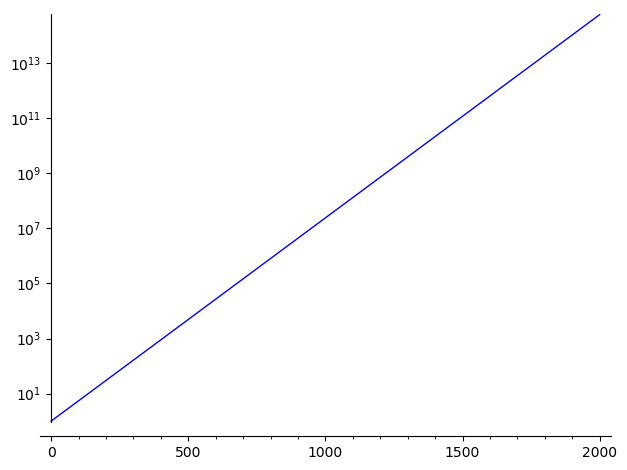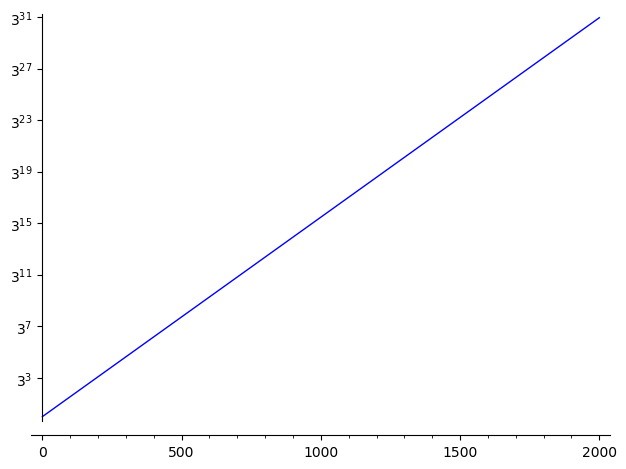2019-03-22 14:41:27 -0500 commented question Sage and pygraphviz help ok, let me ask Iguananaut to take a look 2019-03-22 14:39:08 -0500 commented question Using sagemath on windows 10 : impossible to attach file still, your question makes sense for other windows users 2019-02-07 18:19:49 -0500 received badge ● Nice Answer (source) 2019-02-07 13:24:27 -0500 answered a question jupyter tutorial Regarding question 2, I also recommend the User Interface Tour in the Help menu of the Jupyter notebook. Regarding question 1, for a single user, you may list available legacy Sage notebooks: sage --notebook=export --list  and you may export a legacy Sage notebook as a Jupyter notebook: sage --notebook=export --ipynb=Output.ipynb admin:10  This is done automatically if one does: sage --notebook=export  See the following help messages for more information: sage --notebook --help sage --notebook=export --help  Maybe this is useful for the multi-user setup, but I have no experience with such a migration. 2019-02-06 06:31:21 -0500 answered a question Groebner basis computation You may do something like: sage: R = QQ['x,y,z'] sage: R Multivariate Polynomial Ring in x, y, z over Rational Field sage: x,y,z = R.gens() sage: I = ideal(x^5 + y^4 + z^3 - 1, x^3 + y^3 + z^2 - 1) sage: B = I.groebner_basis() sage: B [y^6 + x*y^4 + 2*y^3*z^2 + x*z^3 + z^4 - 2*y^3 - 2*z^2 - x + 1, x^2*y^3 - y^4 + x^2*z^2 - z^3 - x^2 + 1, x^3 + y^3 + z^2 - 1]  This example was taken from the documentation on Ideals in multivariate polynomial rings 2019-02-06 04:41:44 -0500 answered a question How can I avoit the scientific notation on the y-axis? An alternative is to use the log scale on the y-axis: sage: plot(exp(0.017*x), (x,0,2000), scale="semilogy")Note that you may change the base of the logarithm this way: sage: plot(exp(0.017*x), (x,0,2000), scale=("semilogy",3))See documentation of plot? for more information. 2019-02-05 14:38:50 -0500 commented question Sage and pygraphviz help Are you able to sage -pip install another package like sage -pip install jupyterlab ? If you have problem, it may be a more general problem. It not, it means it is specific to pygraphviz. In which case are you? 2019-01-31 13:12:51 -0500 commented question Sage and pygraphviz help I do not have Windows available close to me to test, but on Ubuntu, I do sage -pip install pygraphviz and I get: Collecting pygraphviz Downloading https://files.pythonhosted.org/packages/7e/b1/d6d849ddaf6f11036f9980d433f383d4c13d1ebcfc3cd09bc845bda7e433/pygraphviz-1.5.zip (117kB) 100% |████████████████████████████████| 122kB 192kB/s Installing collected packages: pygraphviz Running setup.py install for pygraphviz ... done Successfully installed pygraphviz-1.5  2019-01-23 08:26:31 -0500 received badge ● Good Answer (source) 2019-01-23 01:57:58 -0500 answered a question How do I save a rational matrix for Mathematica and Matlab to read? You want to use the method _mathematica_init_(). For matlab, I haven't seen _matlab_init_() method but there is a _octave_init_() method and according to Octave website "The Octave syntax is largely compatible with Matlab" : sage: A = sin(x) sage: A._mathematica_init_() 'Sin[x]' sage: A._octave_init_() 'sin(x)' sage: B = matrix(3, range(9)) sage: B._mathematica_init_() '{{0, 1, 2}, {3, 4, 5}, {6, 7, 8}}' sage: B._octave_init_() '[0 1 2]\n[3 4 5]\n[6 7 8]'  You have a bunch of those methods: sage: [method for method in dir(A) if method.endswith('_init_')] ['_axiom_init_', '_fricas_init_', '_gap_init_', '_giac_init_', '_gp_init_', '_interface_init_', '_kash_init_', '_macaulay2_init_', '_magma_init_', '_maple_init_', '_mathematica_init_', '_maxima_init_', '_maxima_lib_init_', '_octave_init_', '_pari_init_', '_polymake_init_', '_r_init_', '_singular_init_']  If you have mathematica installed, you may use it directly from sage by doing things like below. For me it does not work, because I do not have mathematica installed: sage: Bm = mathematica(B) ... TypeError: unable to start mathematica: End Of File (EOF). Exception style platform. sage: Bm.Inverse() # or something like this  Conversely, with sage 8.6 because of ticket #25501 you may do: ┌────────────────────────────────────────────────────────────────────┐ │ SageMath version 8.6, Release Date: 2019-01-15 │ │ Using Python 2.7.15. Type "help()" for help. │ └────────────────────────────────────────────────────────────────────┘ sage: from sage.symbolic.integration.external import symbolic_expression_from_mathematica_string sage: symbolic_expression_from_mathematica_string('Sin[x]') sin(x) sage: symbolic_expression_from_mathematica_string('{{0, 1, 2}, {3, 4, 5}, {6, 7, 8}}') [[0, 1, 2], [3, 4, 5], [6, 7, 8]]  2019-01-23 00:31:29 -0500 received badge ● Nice Answer (source) 2019-01-21 10:16:01 -0500 answered a question Recovering numbers from continued fraction It used to be that continued_fraction could only deal with finite continued fraction expansions. Thanks to work made in 2014 by Vincent Delecroix and other authors/reviewers, Sage deals with ultimately periodic CF expansions (i.e., quadratic irrationals). If you look at the documentation of continued_fraction?, you will see this example: sage: cf = continued_fraction([(1,1),(2,8)]); cf [1; 1, (2, 8)*] sage: cf.value() 2/11*sqrt5 + 14/11  Therefore, to construct the golden ratio as a quadratic number from the CF expansion you do: sage: preperiod = () sage: period = (1,) sage: cf = continued_fraction([preperiod, period]) sage: cf [(1)*] sage: cf.value() 1/2*sqrt5 + 1/2 sage: cf.value().parent() Number Field in sqrt5 with defining polynomial x^2 - 5 sage: cf.value().n() 1.61803398874989  Conversely, starting from a quatratic irrational, you may find the preperiod and period of its CF expansion: sage: K. = NumberField(x^2-13, embedding=3.5) sage: continued_fraction(sqrt13/6+1/5) [0; 1, (4, 43, 30, 43, 4, 3, 2, 42, 1, 4, 1, 1080, 1, 4, 1, 42, 2, 3)*] sage: continued_fraction(sqrt13/6+1/5).preperiod() (0, 1) sage: continued_fraction(sqrt13/6+1/5).period() (4, 43, 30, 43, 4, 3, 2, 42, 1, 4, 1, 1080, 1, 4, 1, 42, 2, 3)  2019-01-09 13:01:43 -0500 received badge ● Notable Question (source) 2019-01-04 05:20:48 -0500 commented answer Quaternion * integer you must do something like: if isinstance(other, Integer): ... return something elif isinstance(other, quater): ... return something else else: raise TypeError('multiplication with other(={}) not defined'.format(other))  2019-01-03 10:31:45 -0500 commented answer Documentation erratum simplify_full exists so that it appears under tab completion on E.simplify. full_simplify exists maybe because sometimes people use this name (in maple?, in mathematica? for instance) 2019-01-03 10:26:41 -0500 answered a question Quaternion * integer by defining a method def __mul__(self, other): in your class called quater. Something like this: def __mul__(self, other): if isinstance(other, Integer): ... return something elif isinstance(other, quater): ... return something else else: raise TypeError('multiplication with other(={}) not defined'.format(other))  2019-01-03 05:20:42 -0500 commented answer Sage Floyd algorithm in Cython Are you welling to add algorithm='Floyd-Warshall-scipy', algorithm='Floyd-Warshall-numpy' to the method shortest_path_all_pairs in Sage and even improve the Sage pure python implementation? That would be awesome. 2018-12-03 03:40:58 -0500 commented answer Error in finding the closest vector in a sublattice of Z^n "one of the main things I want to do is simply orthogonally project a vector onto a subspace spanned by the columns of a matrix, which is functionality Sage also seems not to have built-in anywhere I can find it." Orthogonal projection can be made with multiplication of matrices, and I think that you can multiply matrices in Sage. In the wikipedia page Projection (linear algebra), you will find a formula of the form: sage: P_A = A*(A.transpose()*A).inverse()*A.transpose()  which gives you a matrix which does the orthogonal projection with respect to the column of the matrix A. 2018-12-01 01:55:47 -0500 marked best answer Can make doc use less memory? Running make doc works fine during the night on my computer because I am not using it. But running make doc is really annoying during the day when let's say I want to use firefox at the same time: the computer gets swapping and becomes unusable. 2018-10-25 09:17:20 -0500 commented answer Is there anything in Sage which solves #SAT ? For an small typical example I have, sage: p Boolean Program (maximization, 275 variables, 65 constraints) sage: p.polyhedron(backend=backend) # takes forever  2018-10-24 10:45:27 -0500 received badge ● Nice Question (source) 2018-10-22 10:40:06 -0500 commented answer Is there anything in Sage which solves #SAT ? Thank you for your idea of using integral_points_count. I will compare the speed with dancing links which was the only way I knew to count solutions. 2018-10-19 04:29:29 -0500 asked a question Is there anything in Sage which solves #SAT ? For example using sharpsat or relsat or Dsharp? 2018-10-18 08:04:43 -0500 commented question Change binary Operation in Monoid/Semigroup Rings Maybe you need to create a new category for the algebra you need. Maybe you will find information on how to do this in the four documentation pages in the section Parents/Elements, Categories and algebraic structures of the thematic tutorials. 2018-09-09 13:56:39 -0500 answered a question Is there a sage command to compute complex numbers? I get: sage: z = 1 + I sage: sqrt(1/z) sqrt(-1/2*I + 1/2)  If you want the real and imaginary parts, you may do: sage: s = sqrt(1/z) sage: s.real().simplify() 1/4*2^(3/4)*sqrt(sqrt(2) + 2) sage: s.imag().simplify() -1/4*2^(3/4)*sqrt(-sqrt(2) + 2)  2018-09-03 03:30:23 -0500 received badge ● Nice Answer (source) 2018-09-03 02:15:55 -0500 answered a question Setting relations between variables You may create a dictionary of variables: import itertools S = {} for i,j,k,l in itertools.product([1..4], repeat=4): S[(i,j,k,l)] = var('S_{}{}{}{}'.format(i,j,k,l))  which then allows you to create a loop to set your relations. Alternatively, you may create only the variable S_ijkl in the loop above and add a line for the others: for i,j,k,l in itertools.product([1..4], repeat=4): if j>i or l>k: continue S[(i,j,k,l)] = var('S_{}{}{}{}'.format(i,j,k,l)) S[(j,i,k,l)] = S[(i,j,l,k)] = S[(j,i,l,k)]  2018-08-29 09:10:55 -0500 commented answer Wrong result from integral(log(1+1/(x**2+1)), x, -oo, oo) So where should we fix this? 2018-08-28 07:49:45 -0500 received badge ● Nice Answer (source) 2018-08-28 04:03:40 -0500 asked a question Why .45 // .28 does not work? Taking modulo works with Python float and Sage float: sage: .45r % .28r 0.16999999999999998 sage: .45 % .28 -0.110000000000000  The quotient works with Python float: sage: .45r // .28r 1.0  But why should it not work with Sage float as well? sage: .45 // .28 Traceback (most recent call last): ... TypeError: unsupported operand parent(s) for //: 'Real Field with 53 bits of precision' and 'Real Field with 53 bits of precision'  2018-08-27 15:48:31 -0500 answered a question Wrong result from integral(log(1+1/(x**2+1)), x, -oo, oo) By default, Sage uses Maxima which indeed gets it wrong. sage: integrate(log(1 + 1/(x**2 + 1)), x, -oo, oo, algorithm='maxima') 2*pi - 2*sqrt(2)*pi  Nonetheless, Sympy and Giac both get it right: sage: integrate(log(1 + 1/(x**2 + 1)), x, -oo, oo, algorithm='sympy') -2*pi + 2*sqrt(2)*pi sage: integrate(log(1 + 1/(x**2 + 1)), x, -oo, oo, algorithm='giac') 2*pi*(sqrt(2) - 1)  2018-08-26 13:18:10 -0500 received badge ● Nice Answer (source) 2018-08-22 08:20:07 -0500 answered a question su2 matrix exponentiation Using sympy, it seems to work: sage: a,b,c,d = [a._sympy_() for a in G.list()] sage: from sympy import Matrix as SympyMatrix sage: G_sympy = SympyMatrix([[a,b], [c,d]]) sage: G_sympy_exp = G_sympy.exp() sage: G_sympy_exp.simplify() sage: G_sympy_exp Matrix([ [(-(cos(theta) - 1)*exp(2*I*t) + cos(theta) + 1)*exp(-I*t)/2, I*(I*sin(phi) - cos(phi))*sin(t)*sin(t heta)], [ (-exp(2*I*t) + 1)*exp(I*phi)*exp(-I*t)*sin(theta)/2, ((cos(theta) + 1)*exp(2*I*t) - cos(theta) + 1)*exp(-I *t)/2]])  Then using copy-paste, I am able to construct the matrix G_exp as a Sage object: sage: G_sympy_exp.tolist() [[(-(cos(theta) - 1)*exp(2*I*t) + cos(theta) + 1)*exp(-I*t)/2, I*(I*sin(phi) - cos(phi))*sin(t)*sin(theta)], [(-exp(2*I*t) + 1)*exp(I*phi)*exp(-I*t)*sin(theta)/2, ((cos(theta) + 1)*exp(2*I*t) - cos(theta) + 1)*exp(-I*t)/2]] sage: G_exp = matrix([[(-(cos(theta) - 1)*exp(2*I*t) + cos(theta) + 1)*exp(-I*t)/2, ....: I*(I*sin(phi) - cos(phi))*sin(t)*sin(theta)], ....: [(-exp(2*I*t) + 1)*exp(I*phi)*exp(-I*t)*sin(theta)/2, ....: ((cos(theta) + 1)*exp(2*I*t) - cos(theta) + 1)*exp(-I*t)/2]] ....: ) sage: G_exp [-1/2*((cos(theta) - 1)*e^(2*I*t) - cos(theta) - 1)*e^(-I*t) (-I*cos(phi) - sin(phi))*sin(t)*sin(theta)] [ -1/2*(e^(2*I*t) - 1)*e^(I*phi - I*t)*sin(theta) 1/2*((cos(theta) + 1)*e^(2*I*t) - cos(theta) + 1)*e^(-I*t)]  Note: I was expecting that G._sympy_() would return the equivalent matrix in Sympy but this method does not exist. It would be nice to add it. Also, there must be an easier way to convert the sympy G_sympy_exp back to a sage matrix instead of the copy-paste I am doing above. 2018-08-17 08:59:28 -0500 answered a question How can I compute the orbits of an automorphism group? Let's say that you are considering the automorphism group of a graph. Then, you do: sage: edges = [(0,1), (1,2), (2,3), (3,4), (4,5), (5,3)] sage: G = Graph(edges, format='list_of_edges') sage: group = G.automorphism_group() sage: group Permutation Group with generators [(4,5)] sage: group.orbits() [, , , , [4, 5]]  You may also get both the automorphism group and the orbits from the graph: sage: group, orbits = G.automorphism_group(orbits=True) sage: group Permutation Group with generators [(4,5)] sage: orbits [, , , , [4, 5]]